# Test: Newton's Second Law of Motion (NCERT)

## 15 Questions MCQ Test NCERTs for NEET: Textbooks, Tests & Solutions | Test: Newton's Second Law of Motion (NCERT)

Description
Attempt Test: Newton's Second Law of Motion (NCERT) | 15 questions in 15 minutes | Mock test for NEET preparation | Free important questions MCQ to study NCERTs for NEET: Textbooks, Tests & Solutions for NEET Exam | Download free PDF with solutions
QUESTION: 1

### Newton's second law of motion is

Solution:

Newton's second law of motion is
F = dp/dt = ma

QUESTION: 2

### Which one of the following statemenls is not true about Newtons second law of motion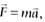Solution:

The second law of motion is a local law which means that force `F` at a point in space (location of the particle) at a certain instant of time is related to a at that instant. Acceleration here and now is determind by the force here and now, not by any history of the motion of the particle.

QUESTION: 3

### The relation, cannot be deduced from Newton's second law, if

Solution:

The relation, can only be deduced from Newton's second law, if mass remains constant with time. If mass depends on time then this relation cannot be deduced.

QUESTION: 4

A large force is acting on a body for a short time. The impulse imparted is equal to the change in:

Solution:

If a large force F acts for a short time dt the impulse imparted I is
I = Fdt = dp/dt dt
I = dp = change in momentum

QUESTION: 5

Which one of the following is not force?

Solution:

Tension, thrust, weight are all common forces in mechanics whereas impulse is not a force.
Impulse = Force x Time Duration

QUESTION: 6

The motion of particle of mass m is given by y = ut + 1/2gt2. The force acting on the particle is

Solution:

Here  y = ut + 1/2gt2
∴ v = dt/dy  = u + gt
Acceleration, a = dt/dv = g
So, the net force acting on the particle is, F = ma = mg

QUESTION: 7

A constant force acting on a body of mass of 5 kg change its speed from 5ms-1 to 10ms-1 in 10s without changing the direction of motion, 'The force acting on the body is

Solution:

Here,m = 5kg, M = 5ms-1, v- 10ms-1, t = 10s, Using v = u + at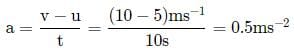As F = ma
∴ F = (5kg)(0.5ms−2) = 2.5N

QUESTION: 8

A bullet of mass 40g moving with a speed of 90 ms-1 enters a heavy wooden block and is stopped after a distance of 60 cm. The average resistive force exerted by the block on the bullet is

Solution:

Here, u = 90  ms−1, v = 0
m = 40gm = 4/1000kg = 0.04 kg, s = 60 cm = 0.6 m
Using v− u2  = 2as
∴ (0)2 − (90)2  = 2a × 0.6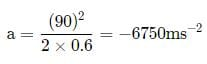∴ The average resistive force exerted by block on the bullet is
F = m × a = (0.04kg)(6750ms−2) = 270N

QUESTION: 9

A body of mass 5kg starts from the origin with an initial velocity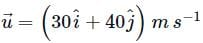. If a constant force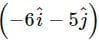N acts on the body, the time in which the y -component of the velocity becomes zero is

Solution:

Here m = 5kg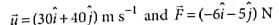∴ uy = 40 m s-1 and Fy = -5 N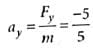= 1 m s-1 vy = 0
As vy = uy + ayt or 0 = 40-1 x t
∴  t = 40s

QUESTION: 10

A body under the action of a force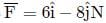acquiring an acceleration of 5ms−2. The mass of the body is

Solution:

Given:and a = 5ms−2
∴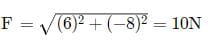Mass of the body is, m = F/a
= 10N / 5ms−2
= 2 kg

QUESTION: 11

A constant retarding force of -50 N is applied to a body of mass 10 kg moving initially with a speed of 10 m s-1.The body comes to rest after

Solution:

Here, F = -50 N (-ve sign for retardation) m=10 kg, u = 10 ms−1, v = 0
As F = ma
∴ a =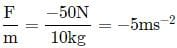Using v = u + at
∴ t =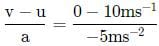= 2 s

QUESTION: 12

A body of mass 0.4 kg starting at origin at t = 0 with a speed of 10 m s-1 in the positive x-axis direction is subjected to a constant F = 8 N towards negative x-axis. The position of the body after 25 s is

Solution:

Mass of the particle, m = 0.4 kg
F = -8 N (minus sign for direction of force)
∴ Acceleration, a = F/m =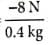= -20 m s-2
The position of the body at any time t is given by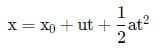The position of the body at t = 0 is 0, therefore x0 = 0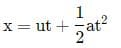Position of the body at t = 25 s
Here, u = 10ms−1, a = −20ms−2, t = 25s
∴ x = 10  × 25 + 1/2(−20)(25)2
= 250 − 6250 = −6000m

QUESTION: 13

If the force on a rocket, moving with a velocity of 300 m s-1 is 210 N, then the rate of combustion of the fuel is

Solution:

Force = d/dt(momemtum)
= d/dt(mv) = v(dm/dt) ⇒ 210 = 300(dm/dt)
rate of combustion, dm/dt = 210/300 = 0.7 kgs-1

QUESTION: 14

A ball of mass m strikes a rigid wall with speed u and rebounds with the same speed. The impulse imparted to the ball by the wall is

Solution:

The situation is as shown in the figure.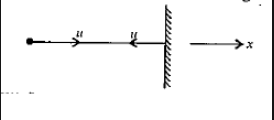pinitial = mu,pfinal = −mu
Impulse imparted to the ball = change in momentum = pinitial = mu,pfinal
= −mu − mu = −2mu

QUESTION: 15

A batsman hits back a ball of mass 0,15 kg straight in the direction of the bowler without changing its initial speed of 12 m s-1. If the ball moves linearly, then the impulse imparted to the ball is

Solution:

Change in momentum = 0.15 x 12 - (-0.15 x 12)
= 3.6 N sUse Code STAYHOME200 and get INR 200 additional OFF Use Coupon Code